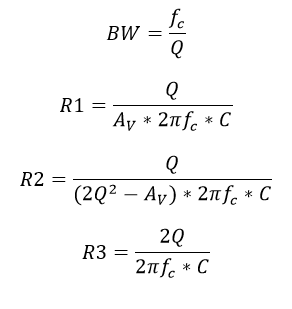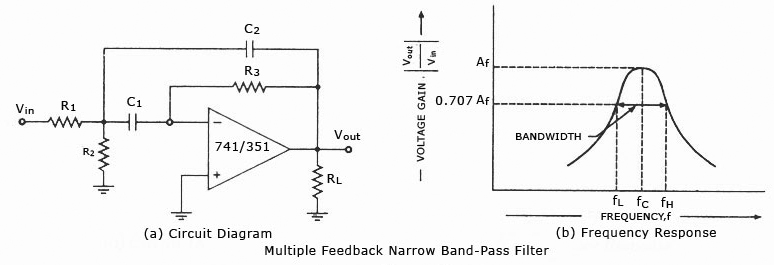# Narrow Band-pass Filter Design Calculator

This CalcTown calculator calculates the resistances, capacitances and bandwidth given the center frequency and quality factor of a narrow band-pass filter.

The advantage of using this multiple feedback active band-pass filter is that one doesn't an inductor (bulky and expensive at low frequencies) to create the band-pass shape. Also, the circuit needs only needs one op-amp device for operation.

#### Result

Hz
Ω
Ω
ΩWhere,

C1, C2 (= C) = Capacitors

R1, R2, R3 = Resistors

Q = Quality Factor of the filter for unit gain

BW = Bandwith of the filter for unit gain

fc = center frequency of the filter for unit gain

An application of this circuit is a graphic equalizer, created by feeding a signal to a number of parallel band-pass filters each tuned to a different frequency; typically octaves apart. One can adjust the strength in each band via. a front panel potentiometer. The outputs of all the filters are then summed to create overall frequency response of the equalizer.